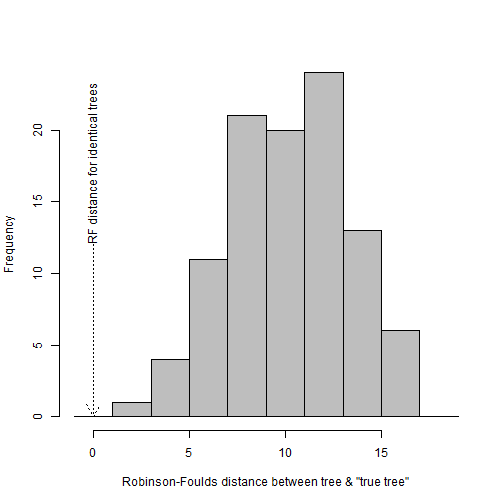## Friday, April 8, 2016

### More on averaging phylogenies in a sample using a criterion of minimum branch-score (Kuhner-Felsenstein) distance

Yesterday I posted about an approach, implemented in phytools, to compute the average tree from a set of trees - defined as the tree (whether included in the set or not) with minimum summed, squared distance to all other trees in the set.

The approach I used yesterday involved the well-known and widely-used tree distance measure called Robinson-Foulds distance. Robinson-Foulds distance is defined (on Wikipedia) as “(A + B) where A is the number of partitions of data implied by the first tree but not the second tree and B is the number of partitions of data implied by the second tree but not the first tree”; however it can also be defined as a sum of the number of edges of the tree that minimum number of edges that need to be added to get from one tree to another.

For instance, to get from:

``````library(ape)
lab4ut="axial",edge.width=2,cex=1.5)
``````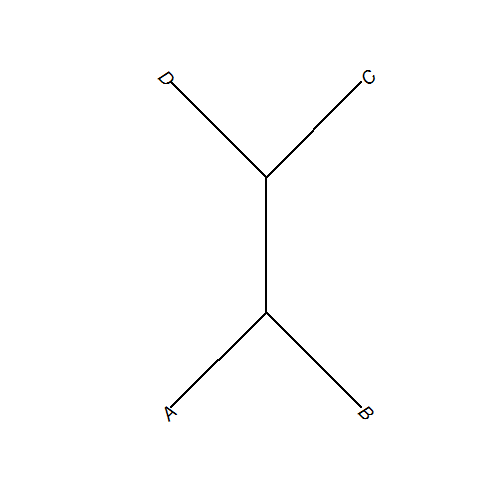to:

``````plot(read.tree(text="((A,D),B,C);"),type="unrooted",
lab4ut="axial",edge.width=2,rotate.tree=-90,cex=1.5)
``````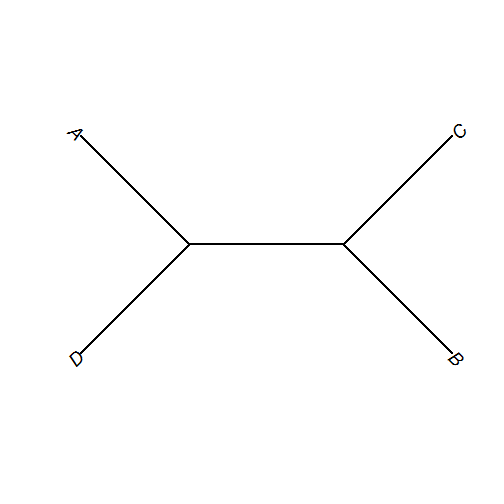one has to first subtract an edge and then create a new one as follows:

``````par(mfrow=c(3,1))
lab4ut="axial",edge.width=2,edge.lty=c(3,1,1,1,1,1),
no.margin=TRUE,cex=2,x.lim=c(-3.4,4.9),y.lim=c(0,2.6))
edge.width=2,no.margin=TRUE,cex=2,x.lim=c(-3.4,4.9),
y.lim=c(-0.2,2.4))
lab4ut="axial",edge.width=2,rotate.tree=-90,cex=2,
edge.lty=c(3,1,1,1,1,1),x.lim=c(-3,5.3),y.lim=c(-0.5,2.1))
``````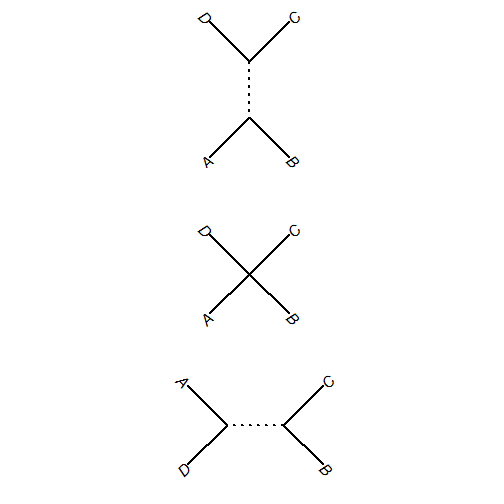resulting in a Robinson-Foulds distance of 2.

Slightly more complicated tree distances also take into account that perhaps adding and subtracting all edges should not be considered equal. For instance, Kuhner-Felsenstein distance (or branch-score-difference) takes into account edge lengths by not merely summing the edges that need to be subtracted or added, but summing the lengths of these edges. This essentially acknowledges that the following:

``````par(mfrow=c(3,1))
lab4ut="axial",edge.width=2,edge.lty=c(3,1,1,1,1,1),
no.margin=TRUE,cex=2,x.lim=c(-1.2,2.6),y.lim=c(-0.2,3.6))
edge.width=2,no.margin=TRUE,cex=2,x.lim=c(-1.2,2.6),
y.lim=c(-1,2.8))
lab4ut="axial",edge.width=2,rotate.tree=-90,cex=2,
edge.lty=c(3,1,1,1,1,1),x.lim=c(-0.3,3.5),y.lim=c(-1.4,2.2))
``````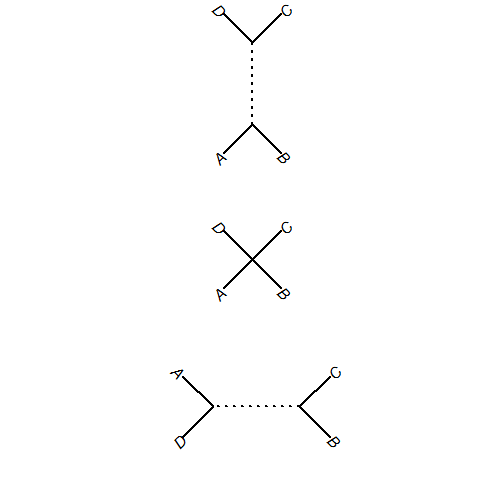is a bigger transformation than the following, in which the internal edge length is very short:

``````par(mfrow=c(3,1))
lab4ut="axial",edge.width=2,edge.lty=c(3,1,1,1,1,1),
no.margin=TRUE,cex=2,x.lim=c(-1.2,2.6),y.lim=c(-0.2,3.6))
edge.width=2,no.margin=TRUE,cex=2,x.lim=c(-1.2,2.6),
y.lim=c(-1,2.8))
lab4ut="axial",edge.width=2,rotate.tree=-90,cex=2,
edge.lty=c(3,1,1,1,1,1),x.lim=c(-1.1,2.7),y.lim=c(-1.4,2.2))
``````We can minimize on this criterion on a set as well. Let's once again use our set of primate phylogenies, as we did yesterday.

The following is quite computationally intensive, unfortunately - but Klaus & I (Klaus in particular for this one) are working on that.

``````library(phytools)
library(phangorn)
bs.tree<-averageTree(trees,method="branch.score.difference")
``````
``````##
##   Function is attempting to find the phylogeny with
##   minimum distance to all trees in set under the
##   "branch score difference" criterion...
``````
``````##   Best SS so far = 6.04071670739487
##   Best SS so far = 6.04071670739487
``````
``````##
##   Solution found after 2 set of nearest neighbor interchanges.
``````
``````plotTree(midpoint(bs.tree))
``````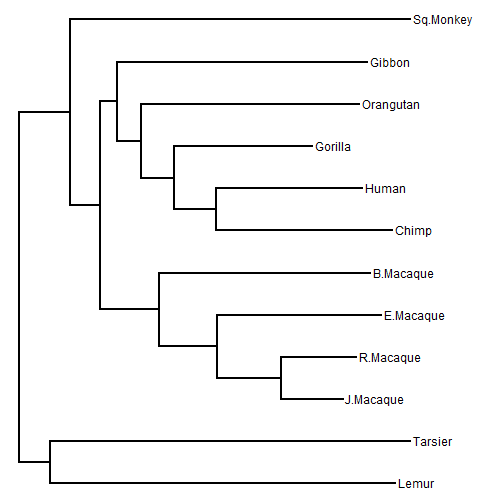One reasonable question might be which, among the set of trees, has the minimum summed distance to all others in the set. We can do that by first computing the all-by-all distances, and then summing the squared columns:

``````D<-sapply(trees,function(x,y) sapply(y,function(y,x)
setNames(treedist(x,y)["branch.score.difference"],NULL),
y=x),y=trees)
d<-colSums(D^2)
obj<-hist(d,breaks=5:20,col="grey",main="",
xlab="sum of squared distances between trees")
arrows(x0=attr(bs.tree,"SQD"),y0=0.5*max(obj\$counts),
x1=attr(bs.tree,"SQD"),y1=0,col="red",lwd=2,length=0.2)
text(x=attr(bs.tree,"SQD"),y=0.5*max(obj\$counts),
``````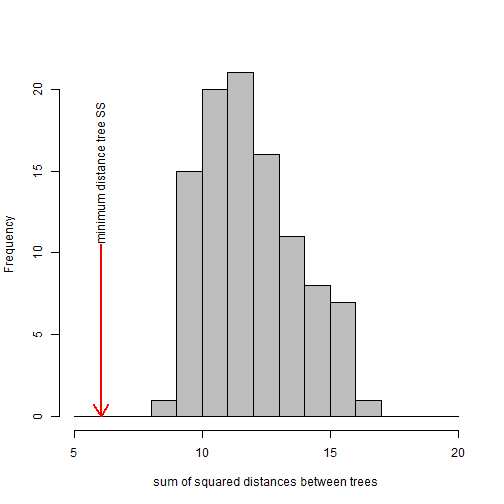This shows that the sum of squared distance from our average tree to all trees in the set is quite a bit smaller than the median or “best” tree in the set, by the same criterion.

We can even show that none of our set of trees has the correct topology, even though the average tree (by this criterion) seems to. We'll do this by computing topological distance (which will be zero when trees are topologically identically) between all trees and the true tree in `bs.tree`.

``````obj<-hist(RF.dist(trees,bs.tree),breaks=0:10*2-1,col="grey",
main="",xlab="Robinson-Foulds distance between tree & \"true tree\"")
arrows(x0=0,y0=0.5*max(obj\$counts),x1=0,y1=0,lwd=1,length=0.2,
lty="dotted")
text(x=0,y=0.5*max(obj\$counts),"RF distance for identical trees",